﻿ Objects > Functions

# Functions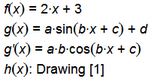A function is a rule that turns input values into output values. For example, the function f(x) = 2·x + 3 is a rule that says “To get an output value, start with the input value, multiply it by 2, and then add 3 to the result.” To evaluate a function you follow the rule for a particular input value. For example, f(5) = 2(5) + 3 = 13.

There are three kinds of functions in Sketchpad:

A symbolically defined function is defined by an algebraic rule.

A derivative function is defined by finding the derivative of another function.

A data-defined function is defined by the top stroke of a drawing or the top edge of a picture.

Once you've created a function, see Using Functions for information on the many ways you can use a function.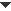Create a Symbolically-Defined Function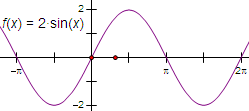To define a new function symbolically, for example, f(x) = 2·sin(x), choose This command opens Sketchpad’s Calculator to allow you to define how the function is calculated. To create a new function and plot it immediately, choose To determine whether the function appears in y= notation or f(x) notation, choose the desired notation from the Calculator’s Equation pop-up menu. See also: How to Use Signum to Construct a Piecewise FunctionCreate a Derivative Function

To create a derivative function, select the function you want to differentiate and choose

The derivative of a symbolically-defined function is usually defined symbolically, as shown on the left. In rare cases of exceptionally complex symbolically-defined functions, the derivative may be defined approximately.

The derivative of a data-defined function (defined by a drawing or picture) is always defined approximately, as shown on the right.

 Symbolic Derivative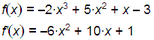Approximate DerivativeTo determine whether the function appears in y= notation or f(x) notation, set the desired notation for the parent function.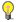Note

 When Sketchpad creates a derivative function, it does not keep track of domain restrictions. For instance, f(x) = ln(x ) has a domain restriction: it's defined for x > 0. Sketchpad shows the correct derivative, f '(x ) = 1/x, but without the domain restriction: the derivative is defined for x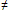0.Create a Data-Defined FunctionTo create a data-defined function based on a drawing or picture, select the drawing or picture and choose The function is defined by the top edge of the drawing or picture. To determine how much smoothing is applied to the function, choose Edit | Properties | Function. An unsmoothed function follows every pixel in the top edge of the drawing or picture; a smoothed function reduces the small variations. To determine whether the function appears in y= notation or f(x) notation, choose